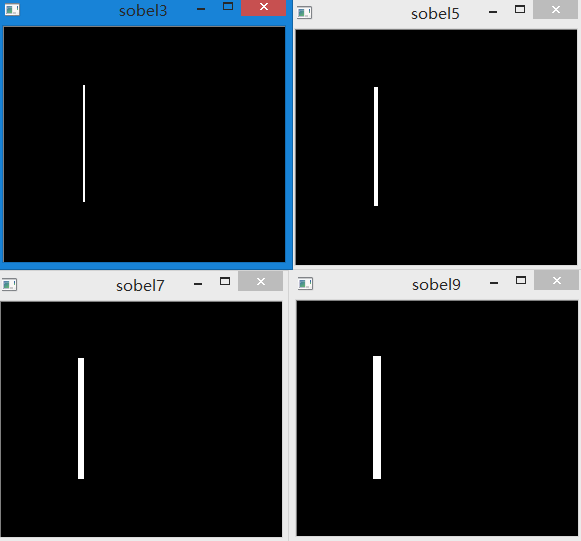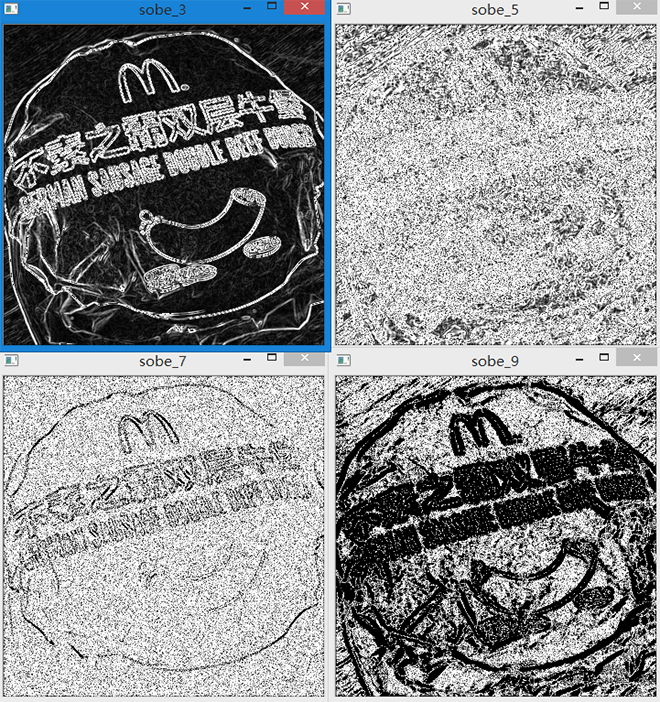# 基于Python的OpenCV图像处理8

## May 20,2017   5475 words   20 min

#### 图像梯度

##### Sobel算子

Sobel算子是高斯平滑与微分操作的结合体，所以它的抗噪声能力很好。 主要用作边缘检测，在技术上，它是离散性差分算子，用来运算图像亮度函数的灰度之近似值。 在图像的任何一点使用此算子，将会产生对应的灰度矢量或是其法矢量。

Sobel算子根据像素点上下、左右邻点灰度加权差，在边缘处达到极值这一现象检测边缘。 对噪声具有平滑作用，提供较为精确的边缘方向信息，边缘定位精度不够高。 当对精度要求不是很高时，是一种较为常用的边缘检测方法。

##### OpenCV实现代码
###### Sobel、Scharr算子实现

# coding=utf-8
import numpy as np
import cv2

gray = cv2.cvtColor(img, cv2.COLOR_BGR2GRAY)

# 第一个参数是待处理图像
# 第二个参数是输出图像深度，-1表示与原图一致
# 第三个参数表示x方向求导，0表示不操作，1表示一阶导数，最高2阶导数
# 第四个参数表示y方向求导，0表示不操作，1表示一阶导数，最高2阶导数
# 第五个参数是卷积核大小，默认为3，-1表示使用Scharr滤波器
sobelx = cv2.Sobel(gray, cv2.CV_64F, 1, 0, ksize=3)

cv2.imshow("sobelx", sobelx)
cv2.imshow("gray", gray)
cv2.waitKey(0)


1.对x方向和y方向求导不能都为0，否则会报错。1,0表示对x方向求导， 0，1表示对y方向求导。而1,1则表示同时对x、y方向求导。 换句话说，即只有某点在x、y方向同时有变化才会显示。演示代码及效果如下：

img = cv2.imread("E:\\520.png")

gray = cv2.cvtColor(img, cv2.COLOR_BGR2GRAY)

sobelx = cv2.Sobel(gray, cv2.CV_64F, 1, 0, ksize=3)
sobely = cv2.Sobel(gray, cv2.CV_64F, 0, 1, ksize=3)
sobel = cv2.Sobel(gray, cv2.CV_64F, 1, 1, ksize=3)

cv2.imshow("gray", gray)
cv2.imshow("sobelx", sobelx)
cv2.imshow("sobely", sobely)
cv2.imshow("sobel", sobel)
cv2.waitKey(0)


2.第二个问题是不同的ksize在图像上最直接的反映就是边缘的粗细。卷积核越大，其对边界越敏感。以下是对比图。可以看出，ksize分别是3、5、7、9的边缘粗细。因为卷积核越大越敏感，所以会检测出更多边界，所以边缘就会粗了。 下面是一幅真实图片的Sobel算子不同卷积核大小的情况。随着卷积核不断增大，图片中的白色也越来越多(也即边界 越来越多),而当卷积核过大之后，反而得不到正确结果了。3.第三个需要注意的是，我们可以通过参数设定输出图像的深度(数据类型)与原图保持一致。但 如果一个从黑道白的边界的导数是正数，而一个从白到黑的边界的导数是负数。如果图像的 深度是uint8时，那么所有的负值都会被截断成0，换句话说即把一半的边界丢失掉。所以解决这个问题 的办法是，将输出的数据类型设置的更高，如cv2.CV_16Scv2.CV_64F等。取绝对值后再把 它们转回到cv2.CV_8U。下面代码演示了这种现象：

img = cv2.imread("E:\\520.png")

gray = cv2.cvtColor(img, cv2.COLOR_BGR2GRAY)

# sobel8u与原图保持一致，所以所有的负数会变为0
sobel8u = cv2.Sobel(gray, -1, 1, 0, ksize=5)

# 首先设置输出的数据类型为64位float
sobelx = cv2.Sobel(gray, cv2.CV_64F, 1, 0, ksize=5)
# 然后对其取绝对值，变成正数
abs_sobelx = np.absolute(sobelx)
# 最后进行类型转换
abs_sobelx_8u = np.uint8(abs_sobelx)

cv2.imshow("gray", gray)
cv2.imshow("sobel8u", sobel8u)
cv2.imshow("abs_sobelx_8u", abs_sobelx_8u)
cv2.waitKey(0)


img = cv2.imread("E:\\5202.png")

gray = cv2.cvtColor(img, cv2.COLOR_BGR2GRAY)

# 拉普拉斯算子，这时的结果是float类型
laplacian = cv2.Laplacian(gray, cv2.CV_64F)
# 对其取绝对值，还是float类型
laplacianf = np.absolute(laplacian)
# 对绝对值转换到uint8
abs_laplacian_8u = np.uint8(laplacianf)

cv2.imshow("gray", gray)
cv2.imshow("laplacian", laplacian)
cv2.imshow("laplacianf", laplacianf)
cv2.imshow("laplacian", abs_laplacian_8u)
cv2.waitKey(0)


4.第四个需要注意的是，如何提取完整边界。通过第三点，我们对第一点的代码进行修正，进行边界提取。

img = cv2.imread("E:\\520.png")

gray = cv2.cvtColor(img, cv2.COLOR_BGR2GRAY)

# x方向
sobelx = cv2.Sobel(gray, cv2.CV_64F, 1, 0, ksize=5)
abs_sobelx_8u = np.uint8(np.absolute(sobelx))

# y方向
sobely = cv2.Sobel(gray, cv2.CV_64F, 0, 1, ksize=5)
abs_sobely_8u = np.uint8(np.absolute(sobely))

# x、y方向
sobelxy = cv2.Sobel(gray, cv2.CV_64F, 1, 1, ksize=5)
abs_sobelxy_8u = np.uint8(np.absolute(sobelxy))

cv2.imshow("gray", gray)
cv2.imshow("sobelx", abs_sobelx_8u)
cv2.imshow("sobely", abs_sobely_8u)
cv2.imshow("sobelxy", abs_sobelxy_8u)
cv2.waitKey(0)


sobel = cv2.add(abs_sobelx_8u, abs_sobely_8u)


##### Laplacian算子

img = cv2.imread("E:\\edge.png")

gray = cv2.cvtColor(img, cv2.COLOR_BGR2GRAY)

# 拉普拉斯算子
# 第一个参数是待处理的图像
# 第二个参数是输出图像的数据类型
# 第三个参数是卷积核的大小，默认为3
laplacian = cv2.Laplacian(gray, cv2.CV_64F, ksize=3)
abs_laplacian_8u = np.uint8(np.absolute(laplacian))

cv2.imshow("gray", gray)
cv2.imshow("laplacian", abs_laplacian_8u)
cv2.waitKey(0)


##### 对比

# coding=utf-8
import numpy as np
import cv2

gray = cv2.cvtColor(img, cv2.COLOR_BGR2GRAY)

# Sobel算子
# x方向
sobelx = cv2.Sobel(gray, cv2.CV_64F, 1, 0, ksize=3)
abs_sobelx_8u = np.uint8(np.absolute(sobelx))
# y方向
sobely = cv2.Sobel(gray, cv2.CV_64F, 0, 1, ksize=3)
abs_sobely_8u = np.uint8(np.absolute(sobely))
# 合并

# Scharr算子
# x方向
scharrx = cv2.Sobel(gray, cv2.CV_64F, 1, 0, ksize=-1)
abs_scharrx_8u = np.uint8(np.absolute(scharrx))
# y方向
scharry = cv2.Sobel(gray, cv2.CV_64F, 0, 1, ksize=-1)
abs_scharry_8u = np.uint8(np.absolute(scharry))
# 合并

# Laplacian算子
laplacian = cv2.Laplacian(gray, cv2.CV_64F, ksize=3)
abs_laplacian_8u = np.uint8(np.absolute(laplacian))

cv2.imshow("gray", gray)
cv2.imshow("laplacian", abs_laplacian_8u)
cv2.imshow("sobel", sobel)
cv2.imshow("scharr", scharr)
cv2.waitKey(0)